# Study on vibration characteristics of rolling mill based on vibration absorber

## Jialei Jiang1, Shuang Liu2, Bin Liu3, Yinghui Wang4, Huifeng Lu5

1, 2, 3, 4, 5School of Electrical Engineering, Yanshan University, Qinhuangdao, China

2Corresponding author

Mathematical Models in Engineering, Vol. 5, Issue 2, 2019, p. 64-72. https://doi.org/10.21595/mme.2019.20871
Received 10 June 2019; accepted 27 June 2019; published 30 June 2019

Copyright © 2019 Jialei Jiang, et al. This is an open access article distributed under the Creative Commons Attribution License, which permits unrestricted use, distribution, and reproduction in any medium, provided the original work is properly cited.
Views 427
Abstract.

The vertical vibration often occurs during the rolling production, which has an influence on the accuracy of rolling mill. In order to effectively suppress the vertical vibration of the rolling equipment, the rolling mill model with vibration absorber device was established. Based on the main resonance singularity of the rolling mill system, the best combination of opening parameters was obtained. The best combination of opening parameters helps the rolling mill system work in a stable area. Finally, the effects of different vibration absorber parameters on the vibration characteristics of the rolling mill system were analyzed. Results show that the vibration absorber device can effectively improve the stability of the rolling mill system.

Highlights
• The rolling mill model with a vibration absorber was established.
• The main resonance singularity of the rolling mill with a vibration absorber was studied.
• The parameters of the vibration absorber seriously affect the stability of the rolling mill.

Keywords: vibration absorber, rolling mill, opening parameters, parameters optimization.

#### 1. Introduction

With the advancement of technology, high-precision rolling products are becoming more and more significant in intelligent manufacturing. However, the vertical vibration of the rolling mill has seriously affected the quality of the rolled products [1-3]. The higher the rolling speed, the more significant the vertical vibration of the rolling mill. When the rolling speed of the rolling reaches the critical value of the rolling speed, which damage the mill equipment [4-6]. Therefore, the vertical vibration not only affects the precision of the rolled products, but also damages the rolling equipment, especially vibration mark in the roll surface.

In recent years, vertical vibration problem of rolling mill is getting more and more attention. Some researchers studied the vertical vibration behavior of rolling mills from different angles, and proposed some effective methods by numerical simulation and physical model. For instance, Fan et al. not only field tested the vibration frequency of CSP (Compact Strip Production) hot tandem mill and its natural frequency, but also obtained the mutual influence relationship between the vibration frequency of hot tandem mill and its natural frequency . Lemma et al. studied a numerical simulation experiment on the physical model of the rolling mill. Then, they predicted the life of rolls by analyzing the simulated value of the rolling mill system . Brusa et al. investigated the vibration behavior of the Sendzimir mill. In order to effectively suppress the torsional vibration and vertical vibration behavior of the rolling mill, they developed a virtual numerical simulator in the Matlab/Simulink environment . Soon after, Liu et al. set up a nonlinear vertical vibration physical model of the mill equipment, and the vertical vibration characteristics of rolling mill under nonlinear restraint force are studied . They analyzed the relationship of mark spacing and vibration source. Finally, they found a way to suppress vertical vibration of rolling mill.

Also, a large number of simulation experiments and physical models have been showed on the vertical vibration behavior. Simultaneously, various mechanical parameters affected the vertical vibration of the rolling mill are also analyzed. Sun et al. discussed the vertical vibration characteristics of the rolls under different load forces and found the distribution pressure of the rolling products seriously affects the vibration behavior of the rolling mill . Zhang et al. researched the vibration behavior of the rolling mill caused by the deformation of the rolled product and the electromechanical coupling model of the rolling mill was established. Meanwhile, they also came up with the cause of the unsteady vibration of the mill . Yang et al. investigated the influence of the roll process parameters on the vertical vibration of the mill. The results show that the nonlinear spring force and nonlinear friction seriously affect the stability of the mill system . Fujita et al. applied electrical discharge coating as a means of improving the wear resistance of the roll surface. Finally, the influence of the electrical machining conditions on the layer thickness, hardness, and surface roughness was evaluated and discussed . Yang Xu et al. took into account the interaction between the roller and the rolled piece and established a vertical vibration model of roll system based on the dynamic friction equation of roll gap .

However, high quality steel is becoming more and more significant in the field of machine manufacturing, but the vibration problem still affects the quality of rolled products. In order to more effectively suppress the vertical vibration of the rolling mill, some scholars began to study the rolling mill system from the control theories. Ling et al. added lateral hydraulic cylinder in horizontal direction of the roller system bearing seats to suppress horizontal vibration of the rolling mill. But this method is likely to have occurrence of primary resonance and super-harmonic resonance . Yan. applied the designing second order torsional vibration observer in the main drive control system. Ultimately, torsional vibration could be restrained by the main drive system of the second order . Liu Shuang et al. established the dynamic equation of some nonlinear torsional vibration system with two masses. After adding the method of the adaptive continuous perturbation control, the amplitude of the system decreases, and there is a transformation from chaotic motion to periodic motion .

Actually, it was difficult to effectively suppress the vertical vibration of the rolling mill by the control algorithm and optimization of the rolling parameters [19, 20]. Although many researchers have suppressed the vertical vibration of the rolling mill by the control algorithm, they did not give a detailed description. Therefore, rolling mill system model with a vibration absorber was established, which relies on the interaction relationship between the rolling mill roller system and the vibration absorber device. The main resonance singularity of the system was analyzed. In addition, we also chose the appropriate combination of opening parameters, which enables the rolling mill system work in a stable area. Finally, the effect of the mass, spring force and friction of the vibration absorber on the vibration behavior of the rolling mill was tested. These provided a reliable basis for the vertical vibration of the rolling mill.

#### 2. A mill rolls model with vibration absorber device

The influence of nonlinear spring force of hydraulic cylinder system on vibration characteristics of the rolling mill system was studied, on the basis of the viewpoint of nonlinear dynamics . The hydraulic cylinder is distributed between the rack and the upper backup roll. Different thickness of plate strips are rolled out through changing the gap size of the two working rolls. The structure of four-roll mill system is shown in Fig. 1.

The characteristics of the upper roll system are the same with the lower roll system due to the symmetry of the four-high mill structure. Therefore, we only analyzed the upper roll system of the rolling mill for the sake of research. In this paper, a physical model of the rolling mill system under the constraints of nonlinear spring force is established. The vibration model of mill rolls is shown in Fig. 2.

Where ${m}_{1}$ is the equivalent mass of backup roll and work roll, ${c}_{1}$ is the equivalent damping of rolled piece, ${k}_{1}$ is the equivalent stiffness of the rolled piece, ${F}_{l}=F\mathrm{c}\mathrm{o}\mathrm{s}\left(\omega t\right)$ is equivalent load force, $F$ is external excitation amplitude, $\omega$ is the angular frequency of external excitation. ${F}_{s}\left(x\right)={k}_{1}^{\text{'}}{x}_{1}+{k}_{3}^{\text{'}}{{x}_{1}}^{3}$ is spring force of hydraulic cylinder. ${k}_{1}^{\text{'}}$ is the equivalent stiffness of hydraulic cylinder, ${k}_{3}^{\text{'}}$ is the nonlinear spring force between frame and upper roll system, ${x}_{1}$ is the vibration displacement of backup roll and work roll.

The vibration absorber and the rolling mill roller are connected by the elastic element and damping element of the vibration absorber. The vibration absorber device is installed to the bracket of the rolling mill rolls. The vibration absorber device and the upper roller system constitute a two-degree-of-freedom system. The installation of the vibration absorber device in the rolling mill system is shown in Fig. 3.

Fig. 1. The structure diagram of mill rolls. 1. Rack, 2. Hydraulic cylinder, 3. Backup roll, 4. Roll gap, 5. Work roll, 6. Rolled piece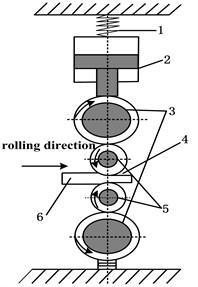Fig. 2. The vibration model of mill rolls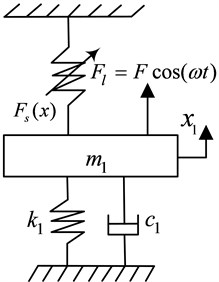Fig. 3. Structure diagram of the rolling mill with vibration absorber. 1. Vibration absorber, 2. Hydraulic cylinder, 3. Backup roll, 4. Upper work roll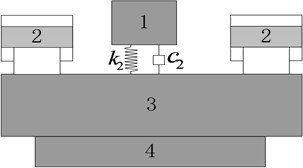The balance position of the rolling mill system and the vibration absorber device are the origin of motion when the system is at a standstill. The size of the vibration displacement of the rolling mill system reflects the vibration intensity. Therefore, in order to control the vertical vibration of the rolling mill system, it is necessary to reduce the vibration displacement the rolling mill system. The vibration energy of the rolling mill system is transferred to the vibration absorber device through the elastic elements and damping elements of the vibration absorber device. The vibration energy of the rolling mill system is mainly divided into two parts. One part is transferred to the friction force of the vibration absorber device. The other part is converted into the kinetic energy of the vibration absorber device. Finally, the vibration displacement of the rolling mill system can effectively be reduced by the vibration absorber device.

Fig. 4. System model of two-degrees-of-freedom with vibration absorber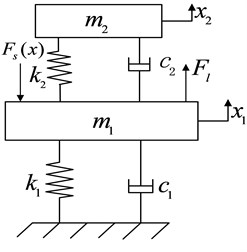Where ${m}_{2}$ is the mass of dynamic vibration absorber, ${x}_{2}$ is the absolute displacement of the vibration absorber, ${k}_{2}$ is the equivalent stiffness of the vibration absorber and rolling mill upper roller system, ${c}_{2}$ is the equivalent stiffness of the vibration absorber and rolling mill upper roller system. On the basis of d’Alembert’s principle . The dynamics balance equation is expressed as:

(1)
$\left\{\begin{array}{l}{m}_{1}{\stackrel{¨}{x}}_{1}+{c}_{1}{\stackrel{˙}{x}}_{1}+{c}_{2}\left({\stackrel{˙}{x}}_{1}-{\stackrel{˙}{x}}_{2}\right)+{k}_{1}{x}_{1}+{k}_{2}\left({x}_{1}-{x}_{2}\right)+\left({k}_{1}^{\mathrm{\text{'}}}+{k}_{3}^{\mathrm{\text{'}}}{{x}_{1}}^{3}\right)=F\mathrm{c}\mathrm{o}\mathrm{s}\left(\omega t\right),\\ {m}_{2}{\stackrel{¨}{x}}_{2}-{c}_{2}\left({\stackrel{˙}{x}}_{1}-{\stackrel{˙}{x}}_{2}\right)-{k}_{2}\left({x}_{1}-{x}_{2}\right)=0.\end{array}\right\$

where ${\stackrel{˙}{x}}_{1}$ is the first derivative of ${x}_{1}$, ${\stackrel{¨}{x}}_{1}$ is the second derivative of ${x}_{1}$, ${\stackrel{˙}{x}}_{2}$ is the first derivative of ${x}_{2}$, ${\stackrel{¨}{x}}_{2}$ is the second derivative of ${x}_{2}$.

#### 3. Solution of two-degree-of-freedom system based on vibration absorber control

Assuming that the rolling mill system is subjected to periodic external load forces, set ${F}_{l}=F\mathrm{c}\mathrm{o}\mathrm{s}\left(\omega t\right)$. By transposition and replacement, Eq. (1) is transformed into a standard form:

(2)
$\left\{\begin{array}{l}{\stackrel{¨}{x}}_{1}+{{\lambda }^{2}}_{1}{x}_{1}=\delta {x}_{2}-\xi \left({\stackrel{˙}{x}}_{1}-{\stackrel{˙}{x}}_{2}\right)-\rho {\stackrel{˙}{x}}_{1}-{k}_{3}^{*}{{x}_{1}}^{3}+{F}_{0}\mathrm{cos}\left(\omega t\right),\\ {\stackrel{¨}{x}}_{2}+{{\lambda }^{2}}_{2}{x}_{2}=\gamma \left({\stackrel{˙}{x}}_{1}-{\stackrel{˙}{x}}_{2}\right)+{\lambda }_{2}{x}_{1}.\end{array}\right\$

where:

Based on the optimal control principle of vibration absorber . ${k}_{2}$ could be approximately expressed as ${k}_{2}=\frac{\mu }{{\left(1+\mu \right)}^{2}}{k}_{1}$, ${c}_{2}$ could be approximately expressed as ${c}_{2}=\frac{\mu }{1+\mu }\sqrt{\frac{3\mu {k}_{1}{m}_{1}}{2\left(1+\mu \right)}}$. $\mu =\frac{{m}_{2}}{{m}_{1}}$ is the ratio of the mass of the vibration absorber and mass of the upper roller system.

Set, $\delta =\epsilon {\delta }_{1}$, $\xi =\epsilon {\xi }_{1}$, $\rho =\epsilon {\rho }_{1}$, ${F}_{0}=\epsilon {F}_{10}$, $\gamma ={\gamma }_{1}$, ${{\lambda }^{2}}_{2}=\epsilon {\omega }_{1}$, ${k}_{3}^{*}=\epsilon {k}_{31}^{*}$.

By parameters replacement, Eq. (2) becomes:

(3)
$\left\{\begin{array}{l}{\stackrel{¨}{x}}_{1}+{{\lambda }^{2}}_{1}{x}_{1}=\epsilon \left[{\delta }_{1}{x}_{2}-{\xi }_{1}\left({\stackrel{˙}{x}}_{1}-{\stackrel{˙}{x}}_{2}\right)-{\rho }_{1}{\stackrel{˙}{x}}_{1}-{k}_{31}^{*}{{x}_{1}}^{3}+{F}_{10}\mathrm{cos}\left(\omega t\right)\right],\\ {\stackrel{¨}{x}}_{2}+{{\lambda }^{2}}_{2}{x}_{2}=\epsilon \left[{\gamma }_{1}\left({\stackrel{˙}{x}}_{1}-{\stackrel{˙}{x}}_{2}\right)+{\omega }_{1}{x}_{1}\right].\end{array}\right\$

In order to get a nonlinear approximate solution of Eq. (2). Set, ${T}_{0}=t$, ${T}_{1}=\epsilon t$. Therefore, the time derivatives are expressed as:

(4)
$\left\{\begin{array}{l}d/dt={D}_{0}+\epsilon {D}_{1}+\dots ,\\ {d}^{2}/d{t}^{2}={{D}_{0}}^{2}+2\epsilon {D}_{0}{D}_{1}+\dots ,\end{array}\right\$

where, ${D}_{n}=\partial /\partial {T}_{n}$, $\epsilon$ is small parameter. On the basis of a multi-scale approach . Set the solution of Eq. (2) as:

(5)
$\left\{\begin{array}{l}{x}_{1}={x}_{11}\left({T}_{0},{T}_{1}\right)+\epsilon {x}_{12}\left({T}_{0},{T}_{1}\right),\\ {x}_{2}={x}_{21}\left({T}_{0},{T}_{1}\right)+\epsilon {x}_{22}\left({T}_{0},{T}_{1}\right).\end{array}\right\$

Substituting Eq. (4) and Eq. (5) into Eq. (3). After Eq. (3) is defined as:

(6)
$\left\{\begin{array}{l}{D}_{0}^{2}{x}_{11}+{\lambda }_{1}^{2}{x}_{11}=0,\\ {D}_{0}^{2}{x}_{21}+{\lambda }_{2}^{2}{x}_{21}=0.\end{array}\right\$
(7)

The solution of Eq. (6) is setting as:

(8)
$\left\{\begin{array}{l}{x}_{11}={A}_{1}\left({T}_{1}\right){e}^{i{\lambda }_{1}{T}_{0}}+{\stackrel{-}{A}}_{1}\left({T}_{1}\right){e}^{-i{\lambda }_{1}{T}_{0}},\\ {x}_{21}={A}_{2}\left({T}_{1}\right){e}^{i{\lambda }_{2}{T}_{0}}+{\stackrel{-}{A}}_{2}\left({T}_{1}\right){e}^{-i{\lambda }_{2}{T}_{0}}.\end{array}\right\$

Substituting Eq. (8) into Eq. (3). On the basis of the small scale detuning parameters, the frequencies are redefined as:$\omega ={\lambda }_{1}+\epsilon \sigma$; ${\lambda }_{2}={\lambda }_{1}+\epsilon {\sigma }_{1}$. Where, $\sigma$ and ${\sigma }_{1}$ are detuning parameters. For avoiding the secular terms of equations, Eq. (7) must meet the conditions as follow:

(9)
$\left\{\begin{array}{l}0.5{F}_{10}{e}^{i\sigma {T}_{1}}+\left({\delta }_{1}+{\xi }_{1}\right){B}_{2}{e}^{i{\sigma }_{1}{T}_{1}}-\left({\xi }_{1}+{\rho }_{1}+2{D}_{1}\right){B}_{1}i{\lambda }_{1}+2i{\lambda }_{1}{D}_{1}{B}_{1}-3{b}_{0}{{B}_{1}}^{2}\overline{{B}_{1}}=0,\\ {\gamma }_{1}{B}_{1}i{\lambda }_{1}{e}^{-i{\sigma }_{1}{T}_{1}}-{\gamma }_{1}{B}_{2}i\omega +{\omega }_{1}{B}_{1}{e}^{-i{\sigma }_{1}{T}_{1}}-2{D}_{1}{B}_{2}i\omega =0.\end{array}\right\$

To solve Eq. (9), we need to express the solution in polar form:

${B}_{1}=0.5a{e}^{i{\phi }_{1}}$, ${B}_{2}=0.5b{e}^{i{\phi }_{2}}$, where, $a$, $b$, ${\phi }_{1}$, ${\phi }_{2}$ both are the functions of ${T}_{1}$. In order to obtain the solution of ${\theta }_{1}={\phi }_{2}-{\phi }_{1}+{\sigma }_{1}{T}_{1}$ equation sets, introducing intermediate variables $\theta$, ${\theta }_{1}$; define that: $\theta =\sigma {T}_{1}-{\phi }_{1}$; Substituting ${B}_{1}$, ${B}_{2}$, $\theta$, ${\theta }_{1}$ into Eq. (9), the modulation equations are expressed as:

(10)

Eliminating $\theta$, ${\theta }_{1}$, the frequency response is obtained in terms of two coupled equations as:

(11)
$\left({\gamma }_{1}a{\lambda }_{1}{\right)}^{2}=\left({\omega }_{1}a{\right)}^{2}+\left({\gamma }_{1}b{\lambda }_{2}{\right)}^{2}+2{\gamma }_{1}b{\lambda }_{2}{\omega }_{1}a\mathrm{s}\mathrm{i}\mathrm{n}{\theta }_{1}+\left[2b{\lambda }_{2}\left(\sigma -{\sigma }_{1}\right){\right]}^{2}.$
(12)

where:

$\mathrm{s}\mathrm{i}\mathrm{n}{\theta }_{1}=\frac{\left({\delta }_{1}+{\xi }_{1}{\right)}^{2}{b}^{2}+\left({\xi }_{1}{\lambda }_{1}a+{\rho }_{1}{\lambda }_{1}a{\right)}^{2}+\left(2{\lambda }_{1}a\sigma {\right)}^{2}-{{F}_{10}}^{2}}{2{a}^{2}b\left({\delta }_{1}+{\xi }_{1}\right)N}$
$\mathrm{c}\mathrm{o}\mathrm{s}{\theta }_{1}=\frac{MN-\left({\delta }_{1}+{\xi }_{1}\right){b}^{2}{\gamma }_{1}{\omega }_{1}{\lambda }_{2}}{4ab{\omega }_{1}\left[{\gamma }_{1}{\lambda }_{1}{\lambda }_{2}+{\lambda }_{2}\left(\sigma -{\sigma }_{1}\right)N\right]}+\frac{L}{4ab{\omega }_{1}\left[{\gamma }_{1}{\lambda }_{1}{\lambda }_{2}+{\lambda }_{2}\left(\sigma -{\sigma }_{1}\right)N\right]\left({\delta }_{1}+{\xi }_{1}\right)},$
$\left\{\begin{array}{l}L={\gamma }_{1}{\lambda }_{1}{\lambda }_{2}{{F}_{10}}^{2}-{\gamma }_{1}{\lambda }_{2}{\omega }_{1}{a}^{2}{N}^{2}-{\gamma }_{1}{\lambda }_{2}{\omega }_{1}\left(2a{\lambda }_{1}\sigma \right),\\ M={{\gamma }_{1}}^{2}{a}^{2}{{\lambda }^{2}}_{1}-{{\omega }_{1}}^{2}{a}^{2}-{{\gamma }_{1}}^{2}{b}^{2}{{\lambda }^{2}}_{2}-4{b}^{2}{{\lambda }^{2}}_{2}\sigma -{\sigma }_{1}{\right)}^{2},\\ N={\xi }_{1}{\lambda }_{1}+{\rho }_{1}{\lambda }_{1}+0.75{k}_{31}^{\mathrm{*}}{a}^{2}.\end{array}\right\$

#### 4.1. Bifurcation characteristic analysis of rolling mill system with vibration absorber

Substituting Eq. (11) into Eq. (12) and eliminating $a$. Then, the bifurcation equation is expressed as:

(13)
${z}^{6}+\alpha {z}^{4}+\beta {z}^{2}+\mu =0.$

where, $\alpha$, $\beta$ are the unfolding parameters linked to the internal parameters of the rolling mill system; $\mu$ is the bifurcation parameter mainly associated with external excitation. On the basis of the Singularity theory, the Eq. (13) is the universal unfolding of the paradigm ${z}^{6}+\mu =0$. Since the equation has many parameters, the bifurcation characteristics cannot be directly displayed on the floor plan. So the rolling mill system with vibration absorber can be divided into three parts and three critical lines. Transition sets of the rolling mill system parameters is shown in Fig. 5.

Fig. 5. Transition sets of system parameters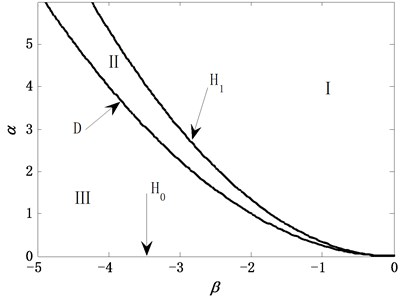Fig. 6. Bifurcation diagram of the system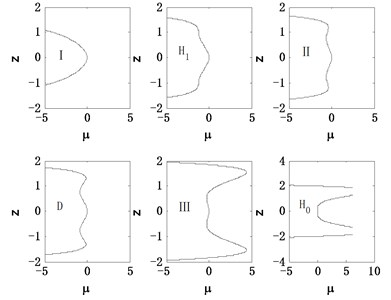As shown in Fig. 6. when opening parameter combination of the rolling mill system is in zone I, the corresponding system bifurcation topology has a stable solution. If the opening parameter combination crosses the critical state of the set ${H}_{1}$, the vibration amplitude of the system with the change of the bifurcation parameters. Meanwhile, the vibration amplitude of the rolling equipment shows unstable multi-value phenomenon when the bifurcation parameter is closed to zero. When the opening parameter from the critical state of the bipolar line point set D to the parameter III area, the multi-valued bifurcation parameter interval of the vibration amplitude of the rolling mill system gradually enlarges. Obviously, the rolling mill vibration system becomes more sensitive in the area III. The opening parameters $\alpha$ and $\beta$ are mainly related to the system cubic coefficient. Therefore, parameters of rolling process should be properly selected. As far as possible, the combination of the numerical values of the opening parameters $\alpha$ and $\beta$ are kept within the range of the region I.

#### 4.2. Internal resonance characteristic analysis

The time-domain diagram and phase diagram under before and after adding the vibration absorber device are shown in Fig. 7 and Fig. 8. When the rolling mill system does not have a vibration absorber device, the vibration displacement is also gradually converge to a steady state. But its convergence time prolonged, and the system phase diagram is also a closed curve. It can be seen that the system is in the relatively steady state, but there is a trend of the unstable development. When the mill system is equipped with a vibration absorber device. The vibration displacement will gradually converge to the steady state with the passage of time, and the system phase diagram is a closed curve. It can be seen that the system is in the steady state.

Fig. 7. The rolling mill system does not have a vibration absorber device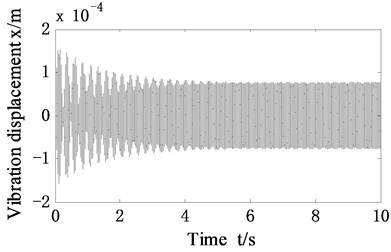a) Time-domain diagram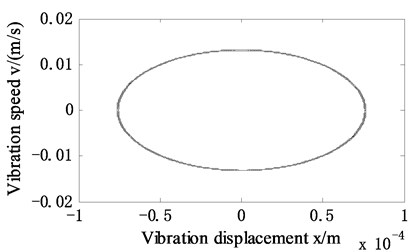b) Phase diagram

Fig. 8. The mill system is equipped with a vibration absorber device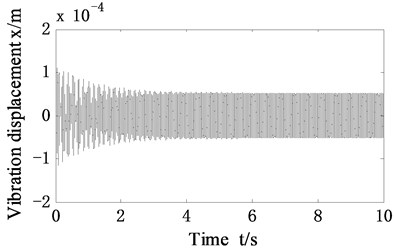a) Time-domain diagram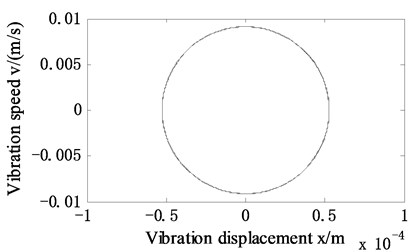b) Phase diagram

From Fig. 9 to Fig. 11, the influence of mass, spring force and friction force of different vibration absorbers on amplitude-frequency characteristic curve of roll mill vibration was analyzed. In Fig. 9, the amplitude-frequency characteristic curves of the vibration absorbers of different masses are different in curvature and height. In Fig. 10, the stiffness coefficient of the vibration absorber can change the curvature of the amplitude-frequency characteristic curve, thereby changing the range of system stability. In Fig. 11, the frictional force of the vibration absorber can change the height of the amplitude-frequency characteristic curve.

Fig. 9. Amplitude frequency characteristics of different absorbers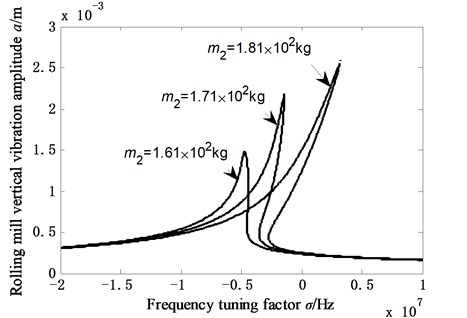Fig. 10. Amplitude frequency characteristics of spring force of different vibration absorbers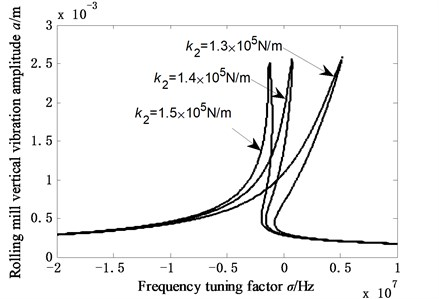Fig. 11. Amplitude frequency characteristics of friction force of different vibration absorbers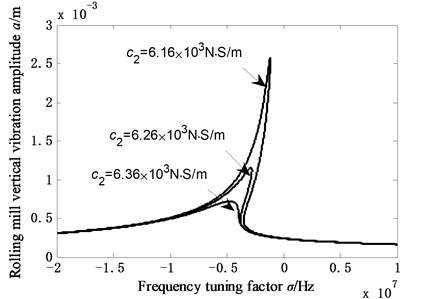#### 5. Conclusions

Taking the impact of vertical vibration of the rolling mill system into consideration, the rolling mill model with a vibration absorber was established. It was found that the vibration energy of the rolling mill system could be transferred to the vibration absorber device. A part of the vibration energy was converted into the heat energy of the vibration absorber device. Another part of the vibration energy was transferred to the kinetic energy of the vibration absorber device. Therefore, the vibration displacement of the rolling mill system is effectively reduced by the vibration absorber device.

On the basis of the main common-amplitude-frequency-response equation, the main resonance singularity of the rolling mill system with a vibration absorber was studied. The differential topology of the rolling mill system showed the stable state of the unique solution, which the parameters combination $\alpha$ and $\beta$ were in the region I. Finally, the best combination of the opening parameters $\alpha$ and $\beta$ were selected by analyzing the system parameter transfer set and the bifurcation topology.

Based on the influence of the mass, spring force and friction force of the vibration absorber on the stability of amplitude-frequency characteristic curve of the rolling mill. Some conclusions are drawn: Within a certain range, the smaller the mass of the vibration absorber device, the smaller the height and curvature of the amplitude-frequency characteristic curve of the rolling mill system. The greater the spring force of the vibration absorber, the smaller the unstable area of the mill system. The greater the friction of the vibration absorber, the smaller the vibration amplitude of the mill system. Therefore, it is important to choose the best mass, spring force and friction of the vibration absorber for effectively suppressing the vertical vibration of the rolling mill system.

1. Niziol J., Swiatoniowski A. Numerical analysis of the vertical vibrations of rolling mills and their negative effect on the sheet quality. Journal of Materials Processing Technology, Vol. 162, Issue 1, 2005, p. 546-550. [Publisher]
2. Heidari A., Forouzan M. R. Optimization of cold rolling process parameters in order to increasing rolling speed limited by chatter vibrations. Journal of Advanced Research, Vol. 4, Issue 1, 2013, p. 27-34. [Publisher]
3. Yarita I., Furukawa K., Seino Y. Analysis of chattering in cold rolling for ultrathin gauge steel strip. Transactions of the Iron and Steel Institute of Japan, Vol. 18, Issue 1, 1978, p. 1-10. [Publisher]
4. Wu S., Shao Y., Wang L., et al. Relationship between vibration marks and rolling force fluctuation for twenty-high roll mill. Engineering Failure Analysis, Vol. 55, Issue 1, 2015, p. 87-99. [Publisher]
5. Yildiz S. K., Forbes J. F., Huang B., et al. Dynamic modeling and simulation of a hot strip finishing mill. Applied Mathematical Modeling, Vol. 33, Issue 7, 2009, p. 3208-3225. [Publisher]
6. Kim Y., Kim C. W., Lee S., et al. Dynamic modeling and numerical analysis of a cold rolling mill. International Journal of Precision Engineering and Manufacturing, Vol. 14, Issue 3, 2013, p. 407-413. [Publisher]
7. Fan X. B., Zang Y., et al. Vibration of CSP hot strip mill. Journal of Mechanical Engineering, Vol. 43, Issue 8, 2007, p. 198-201. [Publisher]
8. Brusa. E., Lemma L. Numerical and experimental analysis of the dynamic effects in compact cluster mills for cold rolling. Journal of Materials Processing Technology, Vol. 209, Issue 5, 2009, p. 2436-2445. [Publisher]
9. Brusa E., Lemma L., Benasciutti D. Vibration analysis of a Sendzimir cold rolling mill and bearing fault detection. Proceedings of the Institution of Mechanical Engineers, Part C: Journal of Mechanical Engineering Science, Vol. 224, Issue 8, 2010, p. 1645-1654. [Search CrossRef]
10. Liu F., Liu B., Liu H., et al. Vertical vibration of strip mill with the piecewise nonlinear constraint arising from hydraulic cylinder. International Journal of Precision Engineering and Manufacturing, Vol. 16, Issue 9, 2015, p. 1891-1898. [Publisher]
11. Sun J. L., Peng Y., Gao Y. A., et al. Simulation and experimental study of horizontal vibration of hot strip mill. Journal of Central South University, Vol. 46, Issue 12, 2015, p. 4497-4503. [Search CrossRef]
12. Zhang Y., Yan X., Ling Q. Electromechanical coupling vibration of rolling mill excited by variable frequency harmonic. Advanced Materials Research, Vol. 912, Issue 914, 2014, p. 662-665. [Publisher]
13. Yang X., Tong C. N., Meng J. J. Rolling force model of vibration zone in cold strip mill. Journal of Vibration, Testing and Diagnosis, Vol. 30, Issue 4, 2010. [Search CrossRef]
14. Fujita N., Kimura Y., Kobayashi K., et al. Dynamic control of lubrication characteristics in high speed tandem cold rolling. Journal of Materials Processing Technology, Vol. 229, 2016, p. 407-416. [Publisher]
15. Yang X., Li Q., Tong C., et al. Vertical vibration model for unsteady lubrication in rolls-strip interface of cold rolling mills. Advances in Mechanical Engineering, Vol. 4, Issue 12, 2012, p. 1432-1437. [Search CrossRef]
16. Ling Q. H., Yan X. Q., Zhang Y. F. Research on nonlinear horizontal vibration suppression of hot strip mill. Journal of Chang’an University (Natural Science), Vol. 35, Issue 6, 2015, p. 145-151. [Search CrossRef]
17. Yan X. Q. Electro-hydraulic coupling vibration control of hot strip mill. Journal of Mechanical Engineering, Vol. 47, Issue 17, 2011, p. 61-65. [Publisher]
18. Liu S., Ai H. L., Lin Z. J., et al. Analysis of vibration characteristics and adaptive continuous perturbation control of some torsional vibration system with backlash. Chaos Solitons and Fractals, Vol. 103, 2017, p. 151-158. [Publisher]
19. Zhu Y., Jiang W., Liu S., et al. Research on influences of nonlinear hydraulic spring force on nonlinear dynamic behaviors of electro-hydraulic servo system. China Mechanical Engineering, Vol. 26, Issue 8, 2015, p. 1085-1091. [Search CrossRef]
20. Han M., Romanovski V. G., Zhang X. Equivalence of the Melnikov function method and the averaging method. Qualitative Theory of Dynamical Systems, Vol. 15, Issue 2, 2016, p. 471-479. [Publisher]
21. Wang Z., Tian Q., Hu H., et al. Nonlinear dynamics and chaotic control of a flexible multibody system with uncertain joint clearance. Nonlinear Dynamics, Vol. 86, Issue 3, 2016, p. 1571-1597. [Publisher]
22. Glocker C. H. Discussion of d’Alembert’s principle for non-smooth unilateral constraints. ZAMM Journal of applied mathematics and mechanics: Zeitschrift für angewandte Mathematik und Mechanik, Vol. 79, 1999, p. 91-94. [Publisher]
23. Guohua Z., Aiguo C., Zhen W., et al. Analysis of lightweight composite body structure for electrical vehicle using the multiscale approach. Journal of Mechanical Engineering, Vol. 52, Issue 6, 2016, p. 145-152. [Publisher]
24. Li L. Optimal parameters selection and engineering implementation of dynamic vibration absorber attached to boring bar. INTER-NOISE and NOISE-CON Congress and Conference Proceedings, 2016. [Search CrossRef]

#### Cited By

 PROCEEDINGS OF ADVANCED MATERIAL, ENGINEERING & TECHNOLOGY Aditya Shekhar, Shubham Kumar, Manish Oraon, Manish Kr. Roy 2020